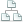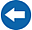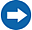Home >Tips >Excel 2016 from Scratch >Calculate sumsSite map

# Excel 2016 from Scratch Exercise Formulas for Calculation

## An Excel tutorial by Peter KalmstromIn this demo in the Excel 2016 from Scratch series Peter Kalmstrom explains how to solve an exercise that contains a combination of formulas used in earlier demonstrations.

We recommend that you try the exercise before you watch the demo. Consider this article a repetition or check-up, and go back and re-view earlier tips in the series if you feel uncertain. Peter uses house costs as an example in this exercise.

### Exercise

The formulas in the demo below has been used earlier in the Excel 2016 from Scratch series, so please try the exercise yourself before you check the solution in the demo. Welcome to download the Excel file Peter uses in the demo and give it a try!

### Content

This is what Peter shows in the demo below:

• How to multiply the value of one cell with the value of another cell.
• How to divide the value of one cell with the value of another cell.
• How to create a formula that calculates sums with the AutoSum button and cell range selection and by selecting each cell that should be included in the sum.
• How to fill formulas with relative references into more cells.
• How to add a currency format to cells.
• How to hide all decimals.
• How to use Cell Styles to differentiate between cells with formulas and input cells.
• How to test formulas.
Peter uses Excel 2016 for his demo, but the Excel basics are the same for earlier versions of Excel.